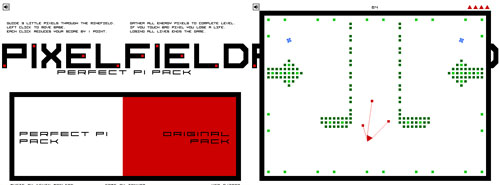# Create a Flash game like PixelField

I spent some time on PixelField and I liked the way you control the “player” and the overall concept.So here I am ready to clone it for Tony Pa’s pleasure…

In this first step I’ll show you how to control the player.

In this version you control a square (in the original one it was a triangle, so don’t say I did’t add something to the original concept… I added a side…) that will move wherever you click the mouse.

Attached to the square there are four little squares that will follow the main square with an elastic effect.

You will find the code familiar if you read Controlling a ball like in Flash Elasticity game tutorial… the four satellites move using this engine.

```// friction and speed_scale
// playing with these variables will affect gameplay
friction = 0.95;
speed_scale = 0.05;
// movieclip where I will draw the four elastics
_root.createEmptyMovieClip("drawing", _root.getNextHighestDepth());
// big square, the "player"
_root.attachMovie("big_square", "big_square", _root.getNextHighestDepth(), {_x:250, _y:200});
//attaching the four satellites
for (x=0; x<=3; x++) {
sq = _root.attachMovie("square", "square_"+x, _root.getNextHighestDepth());
// set their initial speeds to zero
sq.xspeed = 0;
sq.yspeed = 0;
}
// main function, to be executed at every frame
_root.onEnterFrame = function() {
// rotate the big square
big_square._rotation += 2;
for (x=0; x<=3; x++) {
// determining where SHOULD be the x-th satellite without elasticity
should_be_x = big_square._x+80*Math.cos((big_square._rotation+45+90*x)*0.0174532925);
should_be_y = big_square._y+80*Math.sin((big_square._rotation+45+90*x)*0.0174532925);
// determining the distance between the point where it SOULD BE and where it IS
dist_x = (should_be_x-_root["square_"+x]._x)*speed_scale;
dist_y = (should_be_y-_root["square_"+x]._y)*speed_scale;
// adding elasticity... refer to http://www.emanueleferonato.com/2007/09/01/controlling-a-ball-like-in-flash-elasticity-game-tutorial/
_root["square_"+x].xspeed += dist_x;
_root["square_"+x].yspeed += dist_y;
_root["square_"+x].xspeed *= friction;
_root["square_"+x].yspeed *= friction;
_root["square_"+x]._x += _root["square_"+x].xspeed;
_root["square_"+x]._y += _root["square_"+x].yspeed;
_root["square_"+x]._rotation = big_square._rotation;
}
// drawing the elastics
drawing.clear();
drawing.lineStyle(1, 0x000000, 50);
drawing.moveTo(big_square._x, big_square._y);
drawing.lineTo(square_0._x, square_0._y);
drawing.moveTo(big_square._x, big_square._y);
drawing.lineTo(square_1._x, square_1._y);
drawing.moveTo(big_square._x, big_square._y);
drawing.lineTo(square_2._x, square_2._y);
drawing.moveTo(big_square._x, big_square._y);
drawing.lineTo(square_3._x, square_3._y);
};
// moving the big square if the player pressed mouse button
_root.onMouseDown = function() {
big_square._x = _root._xmouse;
big_square._y = _root._ymouse;
};```

Enjoy... press the mouse and see what happens

214 GAME PROTOTYPES EXPLAINED WITH SOURCE CODE
// 1+2=3
// 10000000
// 2 Cars
// 2048
// Avoider
// Ballz
// Block it
// Blockage
// Bloons
// Boids
// Bombuzal
// Breakout
// Bricks
// Columns
// CubesOut
// Dots
// DROP'd
// Dudeski
// Eskiv
// Filler
// Fling
// Globe
// HookPod
// Hundreds
// InkTd
// Iromeku
// Lumines
// Magick
// MagOrMin
// Maze
// Memdot
// Nano War
// Nodes
// o:anquan
// Ononmin
// Pacco
// Phyballs
// Platform
// Poker
// Pool
// Poux
// Pudi
// qomp
// Racing
// Renju
// SameGame
// Security
// Sling
// Slingy
// Sokoban
// Splitter
// Sproing
// Stack
// Stringy
// Sudoku
// Tetris
// Threes
// Toony
// Turn
// TwinSpin
// vvvvvv
// Wordle
// Worms
// Yanga
// Zhed
// zNumbers